# Search

About 10 Search Results Matching Types of Worksheet, Worksheet Section, Generator, Generator Section, Subjects matching Geometry, Type matching Worksheet, Grades matching 5th Grade, Similar to Halloween Worksheets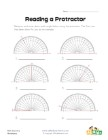## Reading a Protractor Worksheet 2

Read each protractor and write down the angles the...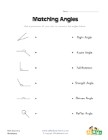## Matching Angles Worksheet

Draw a line to match each of the angles with the t...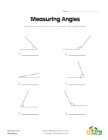## Measuring Angles Worksheet 1

Measure each of the angles with a protractor and w...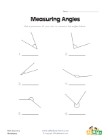## Measuring Angles Worksheet 2

Use a protractor to measure each of the angles.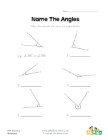## Name the Angles Worksheet

Follow the example and name each of the angles.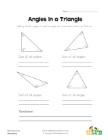## Angles in a Triangle Worksheet

Kids learn that the angles of a triangle always ad...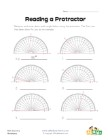## Reading a Protractor Worksheet 1

Read each protractor and write down the angles the...